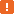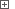# Obtaining Selected Records in Microsoft DataGridWhen testing your application with Microsoft DataGrid .NET controls, you may need to select multiple rows in a grid. Then, you may need to determine which records are selected in the grid in order to process them in a special way.

You can check whether a particular grid row is selected using the grid’s internal `IsSelected` method:

GridObj.IsSelected( RowIndex )

The RowIndex parameter of this method specifies the zero-based index of the row whose selected state you want to get. The method returns True if the row is selected and False otherwise.

To get the indexes of all selected rows in the DataGrid control, you need to iterate through all grid rows and call the `IsSelected` method for each row.In order for TestComplete to have access to internal objects, properties and methods of the DataGrid control, the .NET Application Support and Microsoft Control Support plugins must be installed and enabled.

The following example demonstrates how you can obtain and process the records selected in the DataGrid control.

###### ExampleView description

JavaScript, JScript

function Main ()
{
var p, Grid, RowIndexes, i;
// Obtain the grid object
p = Sys.Process("DataGridSample");
Grid = p.WinFormsObject("Form1").WinFormsObject("dataGrid1");

// Select a range of rows
SelectRange (Grid, 1, 6);

// Get the selected rows' indexes
RowIndexes = GetSelectedRowIndexes (Grid);

// Post the selected rows' cell values to the log
for (i=0; i<RowIndexes.length; i++)
Log.Message (RowIndexes[i] + ": " + Grid.wValue(RowIndexes[i], "Customer Name").ToString().OleValue);
}

function SelectRange (Grid, StartRowIndex, EndRowIndex)
{
// Select the first row of the range
Grid.ClickRowIndicator (StartRowIndex);
// Extend the selection
Grid.ClickRowIndicator (EndRowIndex, skShift);
}

function GetSelectedRowIndexes (Grid)
{
var arr = new Array ();

// Iterate through all grid rows
for (var i=0; i<Grid.wRowCount; i++)
// If the row is selected, ...
if (Grid.IsSelected(i))
// ... save its index to the array
arr.push (i);

return arr;
}

Python

``````def Main ():
# Obtain the grid object
p = Sys.Process("DataGridSample")
Grid = p.WinFormsObject("Form1").WinFormsObject("dataGrid1")

# Select a range of rows
SelectRange (Grid, 1, 6)

# Get the selected rows' indexes
RowIndexes = GetSelectedRowIndexes (Grid)

# Post the selected rows' cell values to the log
for i in range(0, RowIndexes.length-1):
Log.Message (RowIndexes[i] + ": " + Grid.wValue(RowIndexes[i], "Customer Name").ToString().OleValue)

def SelectRange (Grid, StartRowIndex, EndRowIndex):
# Select the first row of the range
Grid.ClickRowIndicator (StartRowIndex)
# Extend the selection
Grid.ClickRowIndicator (EndRowIndex, skShift)

def GetSelectedRowIndexes (Grid):
arr = list()

# Iterate through all grid rows
for i in range(0, Grid.wRowCount):
# If the row is selected, ...
if Grid.IsSelected[i]:
# ... save its index to the array
arr.push (i)

return arr``````

VBScript

Sub Main
Dim p, Grid, RowIndexes, i

' Obtain the application process and the grid object
Set p = Sys.Process("DataGridSample")
Set Grid = p.WinFormsObject("Form1").WinFormsObject("dataGrid1")

' Select a range of rows
Call SelectRange (Grid, 1, 6)

' Get the selected rows' indexes
RowIndexes = GetSelectedRowIndexes (Grid)

' Post the selected rows' cell values to the log
For i = 0 To UBound(RowIndexes)
Log.Message (RowIndexes(i) & ": " & Grid.wValue(RowIndexes(i), "Customer Name").ToString.OleValue)
Next
End Sub

Sub SelectRange (Grid, StartRowIndex, EndRowIndex)
' Select the first row of the range
Grid.ClickRowIndicator (StartRowIndex)
' Extend the selection
Call Grid.ClickRowIndicator (EndRowIndex, skShift)
End Sub

Function GetSelectedRowIndexes (Grid)
Dim arr, i, j

arr = Array ()
' Iterate through the ranges collection
j = 0 ' Counter of Indexes elements

' Iterate through all grid rows
For i = 0 To Grid.wRowCount-1
' If the row is selected, ...
If (Grid.IsSelected(i)) Then
' ... save its index to the array
Redim Preserve arr (UBound(arr) + 1) ' Increase the array size
arr(j) = i
j = j + 1
End If
Next

GetSelectedRowIndexes = arr
End Function

DelphiScript

function GetSelectedRowIndexes (Grid); forward;
procedure SelectRange (Grid, StartRowIndex, EndRowIndex); forward;

procedure Main;
var p, Grid, RowIndexes, i : OleVariant;
begin
// Obtain the grid object
p := Sys.Process('DataGridSample');
Grid := p.WinFormsObject('Form1').WinFormsObject('dataGrid1');

// Select a range of rows
SelectRange (Grid, 1, 6);

// Get the selected rows' indexes
RowIndexes := GetSelectedRowIndexes (Grid);

// Post the selected rows' cell values to the log
for i := 0 to VarArrayHighBound(RowIndexes, 1) do
Log.Message (aqConvert.VarToStr(RowIndexes[i]) + ': ' + Grid.wValue[RowIndexes[i], 'Customer Name'].ToString.OleValue);
end;

procedure SelectRange (Grid, StartRowIndex, EndRowIndex);
begin
// Select the first row of the range
Grid.ClickRowIndicator (StartRowIndex);
// Extend the selection
Grid.ClickRowIndicator (EndRowIndex, skShift);
end;

function GetSelectedRowIndexes (Grid);
var arr, i, j : OleVariant;
begin
arr := CreateVariantArray(-1,-1);
j := 0; // Counter of arr elements

// Iterate through all grid rows
for i := 0 to Grid.wRowCount-1 do
// If the row is selected, ...
if Grid.IsSelected(i) then
begin
// ... save its index to the array
VarArrayRedim (arr, VarArrayHighBound(arr, 1) + 1); // Increase the array size
arr [j] := i;
j := j + 1;
end;

Result := arr;
end;

C++Script, C#Script

function Main ()
{
var p, Grid, RowIndexes, i;
// Obtain the grid object
p = Sys["Process"]("DataGridSample");
Grid = p["WinFormsObject"]("Form1")["WinFormsObject"]("dataGrid1");

// Select a range of rows
SelectRange (Grid, 1, 6);

// Get the selected rows' indexes
RowIndexes = GetSelectedRowIndexes (Grid);

// Post the selected rows' cell values to the log
for (i=0; i<RowIndexes["length"]; i++)
Log["Message"](RowIndexes[i] + ": " + Grid["wValue"](RowIndexes[i], "Customer Name")["ToString"]()["OleValue"]);
}

function SelectRange (Grid, StartRowIndex, EndRowIndex)
{
// Select the first row of the range
Grid["ClickRowIndicator"](StartRowIndex);
// Extend the selection
Grid["ClickRowIndicator"](EndRowIndex, skShift);
}

function GetSelectedRowIndexes (Grid)
{
var arr = new Array ();

// Iterate through all grid rows
for (var i=0; i<Grid["wRowCount"]; i++)
// If the row is selected, ...
if (Grid["IsSelected"](i))
// ... save its index to the array
arr["push"](i);

return arr;
}